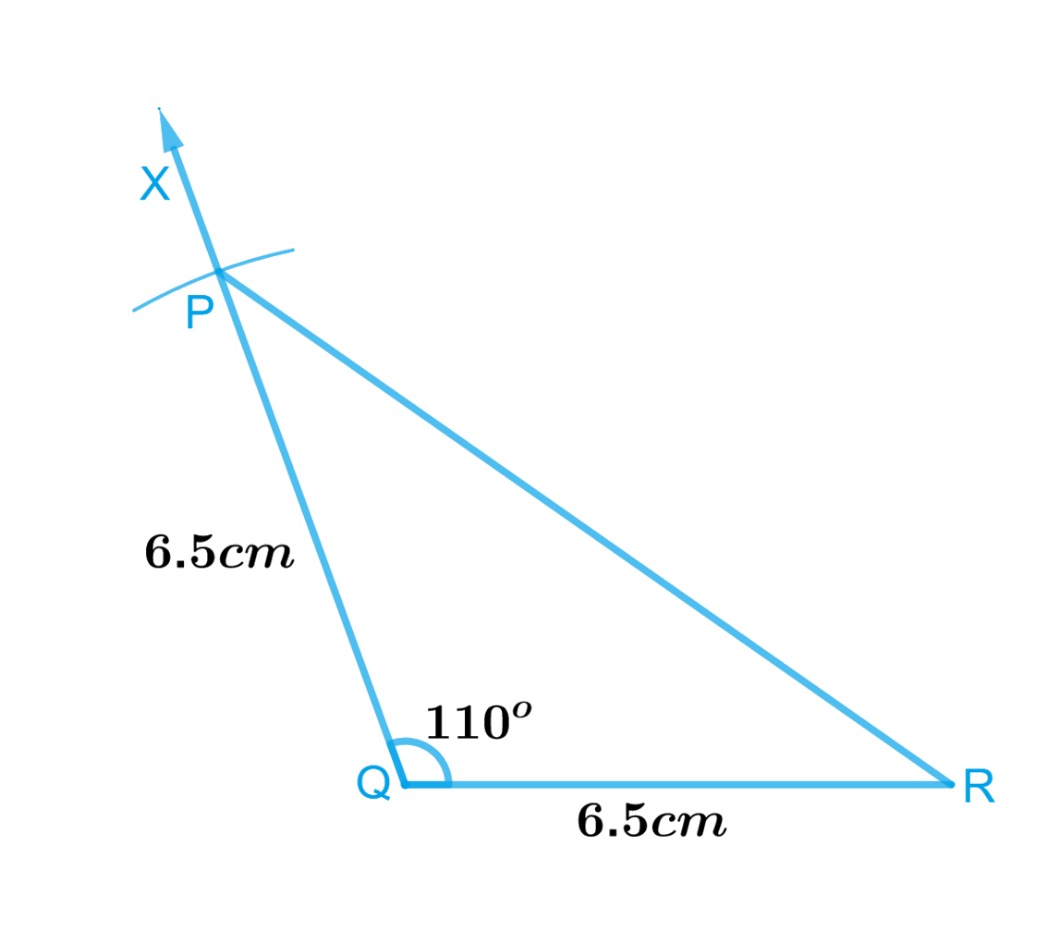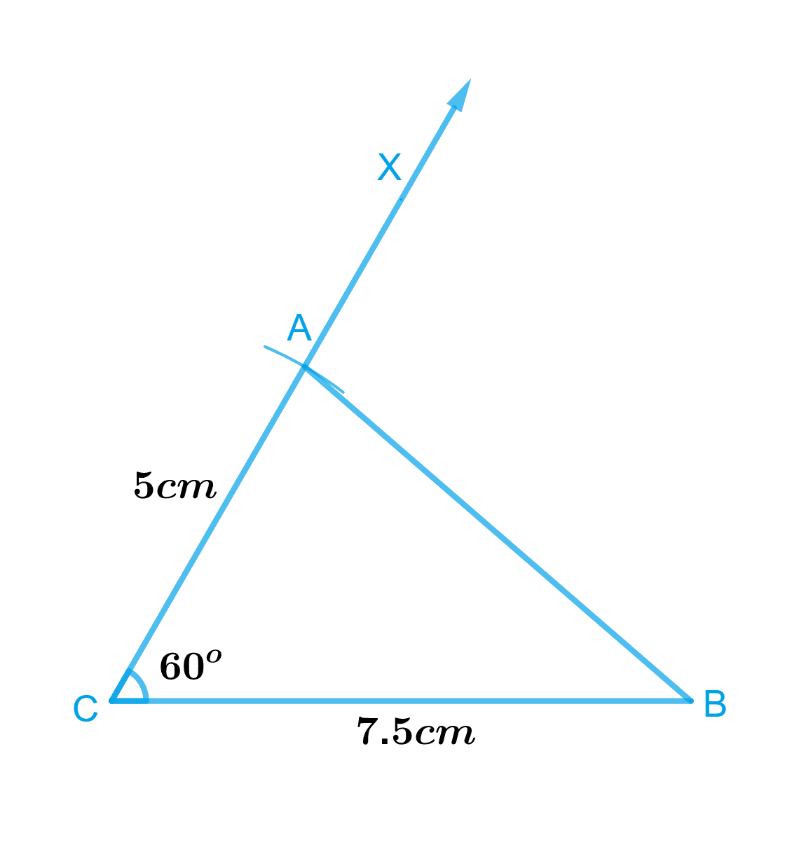# Exercise 10.3 Practical-Geometry -NCERT Solutions Class 7

Go back to  'Practical Geometry'

## Chapter 10 Ex.10.3 Question 1

Construct $$ΔDEF$$ such that $$DE = 5\,\rm{ cm}$$, $$DF = 3\,\rm{ cm}$$ and $$m∠EDF = 90^\circ$$.

### Solution

What is known?

Lengths of sides of a $$ΔDEF$$ and measure of one of the angles.

To construct:

A triangle $$ΔDEF$$ such that $$DE = 5\,\rm{ cm}$$, $$DF = 3\,\rm{ cm}$$ and $$∠EDF = 90^\circ$$.

Reasoning:

To construct a $$ΔDEF$$ first, we draw a rough sketch with the given measure such that $$DE = 5\,\rm{ cm}, DF = 3\,\rm{ cm}$$ and $$∠EDF = 90^\circ$$, then follow the steps given below.

Steps:Steps of construction:

1. Draw a line segment $$DE$$ of length $$5\,\rm{cm}.$$
2. At $$D,$$ draw $$DX$$ making $$90^\circ$$ with $$DE$$.
3. With $$D$$ as centre, draw an arc of radius $$3\,\rm{cm}$$. It cuts $$DX$$ at the point $$F.$$
4. Join $$EF$$ to get the required triangle.

Thus,​​​​​​$$ΔDEF$$  is the required triangle.

## Chapter 10 Ex.10.3 Question 2

Construct an isosceles triangle in which the lengths of each of its equal sides is $$6.5\,\rm{ cm}$$ and the angle between them is $$110^\circ$$

### Solution

What is known?

Lengths of each of its equal sides is $$6.5\,\rm{ cm}$$ and the angle between them is $$110^\circ.$$

To construct:

An isosceles triangle in which the lengths of each of its equal sides is $$6.5\,\rm{ cm}$$ and the angle between them is $$110^\circ.$$

Reasoning:

To construct an isosceles triangle in which the lengths of each of its equal sides is $$6.5\,\rm{ cm}$$ and the angle between them is $$110^\circ,$$  follow the steps given below.

Steps:Steps of construction :

1. Draw a line segment $$QR$$ of length $$6.5\,\rm{cm}.$$
2. At $$Q,$$ draw $$QX$$ making an angle of $$110^\circ$$ with $$QR.$$
3. With $$Q$$ as centre, draw an arc of radius $$6.5\,\rm{cm}.$$ It cuts $$QX$$ at the point $$P.$$
4. Join $$PR$$. Triangle $$PQR$$ is the required isosceles triangle.

## Chapter 10 Ex.10.3 Question 3

Construct $$\Delta ABC$$ with $$BC = 7.5\,\rm{ cm}$$, $$AC = 5\,\rm{ cm}$$ and $$m∠C = 60^\circ$$.

### Solution

What is known?

Lengths of sides of a triangle are $$BC = 7.5\,\rm{ cm}$$, $$AC = 5\,\rm{ cm}$$ and $$∠C = 60^\circ$$.

To construct:

A triangle $$\Delta ABC$$  with $$BC = 7.5\,\rm{ cm}$$, $$AC = 5\,\rm{ cm}$$ and $$∠C = 60^\circ$$.

Reasoning:

To construct a triangle $$ΔABC$$ with $$BC = 7.5\,{\rm{ cm}}, AC = 5\,\rm{ cm}$$ and $$∠C = 60^\circ$$, follow the steps given below.

Steps:Steps of construction :

1. Draw a line segment $$BC$$ of length $$7.5\,\rm{cm}.$$
2. At $$C$$, draw $$CX$$ making $$60^\circ$$ with $$BC$$.
3. With $$C$$ as centre, draw an arc of radius $$5\,\rm{cm}.$$ It cuts $$CX$$ at the point $$A$$.
4. Join $$AB.$$

Triangle $$ABC$$ is the required triangle.

Related Sections
Related Sections
Instant doubt clearing with Cuemath Advanced Math Program